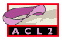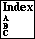MAKE-ORD

a constructor for ordinals.
```Major Section:  PROGRAMMING
```

`Make-ord` is the ordinal constructor. Its use is recommended instead of using `cons` to make ordinals.

`(make-ord fe fco rst)` corresponds to the ordinal (w^fe)fco + rst. Thus the first infinite ordinal, w (omega), is constructed by

```(make-ord 1 1 0)
```
and, for example, the ordinal (w^2)5 + w2 + 7 is constructed by:
```(make-ord 2 5 (make-ord 1 2 7)) .
```

The reason `make-ord` is used rather than `cons` is that it allows us to reason more abstractly about the ordinals, without having to worry about the underlying representation.